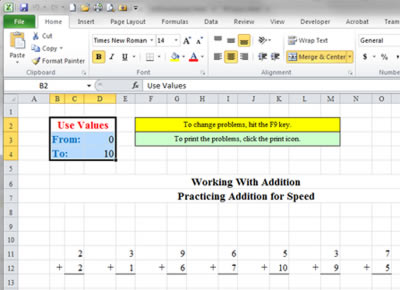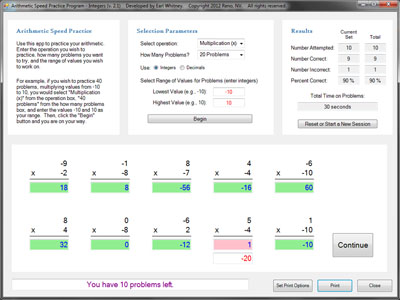"It is not enough to have a good mind. The main thing is to use it well."
- René Descartes

Each link above takes you to a section of this website that contains material developed specifically for the purposes identified.

# Arithmetic Speed Practice

Success in math classes can be enhanced by increasing your ability in arithmetic, both in accuracy and in speed. The Speed Practice files presented on this page are designed to help you do this. All math problems involve arithmetic. Improving your arithmetic skills will allow you to spend more time learning math and less time struggling with arithmetic. This will, hopefully, both improve your grades and make learning math more fun.

There are two Speed Practice files from which you may choose. Each generates problems that can be used to increase a student's speed in arithmetic. The Speed Practice Excel File is a Microsoft Excel file that allows you to create printable pages of problems, but cannot be worked on the computer screen. The second file is a PC app that generates problems to be worked on the computer screen.

## What's In the Excel File?

There are three separate sheets in the Excel file. One sheet generates problems in addition and subtraction. One sheet generates problems in multiplication and division. One sheet generates subtraction problems subtracting from 10 and 100. Each sheet provides 64 problems for each operation on the sheet (a total of 128 problems on each sheet).

## How Does The Excel File Work?In the sheets containing addition, subtraction, multiplication and division problems, you set the limits of what you want to test in the upper left-hand side of the Excel worksheet, and press F9 to generate a new set of random problems.

For example, if you want to practice addition and subtraction of numbers from 0 to 10, you enter 0 and 10 in the "From" and "To" fields in the upper left-hand side of the Excel spreadsheet, and press F9. If you want to practice addition and subtraction of numbers from -25 to 25, you enter -25 and 25 in the "From" and "To" fields in the upper left-hand side of the Excel spreadsheet, and press F9. (Note: you may click on the image above to see a larger illustration of the file.)

If you do not like the random problems that were generated, press F9 again and the spreadsheet will give you a new set of problems. When you have a set of problems you like, click on the Excel print icon to print out both the problems and the answers to the problems.

## How Do the Speed Practice PC Apps Work?If you prefer to work on the screen instead of printing problems and working them out on paper, you may want to download any of the following:

• Speed Practice App version 1.0 – contains problems that can be used to increase a student's accuracy and speed in arithmetic. Uses integers only.
• Speed Practice App version 2.1 – Same as version 1.0, but allows you to use either integers or decimals. Also contains a few other enhancements. Most users should download this version.
• Speed Practice App for Netbooks version 2.1 – Same as Speed Practice App version 2.1, but designed for netbook computers with a maximum screen height of 600 pixels. Uses integers and/or decimals.

To use any of these apps, enter the operation you wish to practice (addition, subtraction, multiplication or division), how many problems you want to try, and the range of values you want to work on; then, click on the "Begin" button to start the program.

For example, if you wish to practice 40 problems, multiplying values from -10 to 10, you would select "Multiplication (x)" from the Select Operation box, "40 problems" from the How Many Problems box, and enter the values -10 and 10 as your range. Then, click the "Begin" button and you are on your way. (Note: you may click on the image above to see a larger illustration of the app.)

The app will provide statistics on your session, including how many problems you got right and wrong, your accuracy percentage and how long it took you to complete the exercise. The time statistic counts only the time you take to do the problems; it does not count the time you take to examine any problems you got wrong. So learn from your mistakes and work to increase your speed. Enjoy!